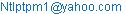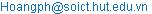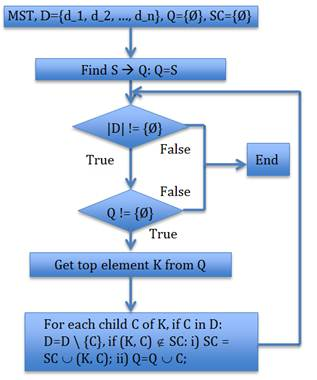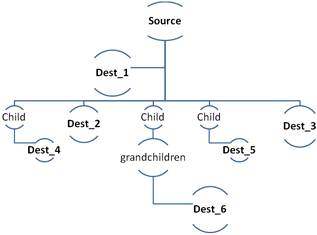Internet of Things and Cloud Computing
Volume 3, Issue 3, October 2015, Pages: 66-73

Innovating Multicast Treeand Artificial Algorithms for Service Based Routing

Nguyen Thanh Long1, Nguyen Duc Thuy2, Pham Huy Hoang3

1Software development division III, Informatics Center of Hanoi Telecommunications, Hoan Kiem, Hanoi, Vietnam

2Center for applied research and technology development, Research institute of Posts and telecommunications, Hanoi, VietNam

3Information technology institute, Ha Noi University of Science Technology, Hanoi, Vietnam(N. T. Long)(N. D. Thuy)(P. H. Hoang)

Nguyen Thanh Long, Nguyen Duc Thuy, Pham Huy Hoang. Innovating Multicast Tree and Artificial Algorithms for Service Based Routing. Internet of Things and Cloud Computing. Vol.3, No. 3, 2015, pp. 66-73. doi: 10.11648/j.iotcc.s.2015030601.18

Abstract: In advance network we may use service Based Routing (SBR), so data is transmitted from a source node to destination nodes are not depended on destination addresses. So, it may be comfortable with new advanced technology as cloud computing and also flexible and reliable. Multicast routing is advanced technique to deliver data simultaneously from one source node to multiple destination nodes with QOS (quality of service). In this paper, we introduce a technique that is extended from multicast technique with multiple multicast trees that are conformed quality of service routing and multiple path routing. It also guarantees QOS of routes such as bandwidth. This technique is based on Greedy, Ant Colony Optimization, artificial neural network and fuzzy logic to get optimal routes to transmit data from one source to multiple destination node very effectively. The usage of the ANT Colony optimization, Greedy, fuzzy logic algorithms to find cyclic or multiple paths routes on each trunk by multiple criterions to transmit data effectively.

Keywords: Manet, Service, Routing

1. Basical Concepts

In order to model a general network in common by a graph in discrete mathematics. In which denote V is the set of vertics that are nodes in the network, E is the set of the edges that are links to connect each pair of nodes in the graph. The routing problem is to make optimization routes from routing table. The inputs of the routing table are collected by routing process. The routing process consists of several minor detail processes: In the reactive or on-demand routing protocol: i) Broadcast packets over network to find routes; ii) Collecting reply packets to build optimal routes; in proactive protocol it usually collects network information through flooding HELLO messages. On receiving HELLO packets, network node updates the routing table to use to find routes as introducing in the next section. HELLO messages are effective to collect network information.

2. Artificial Algorithms

2.1. Fuzzy Logic

The fuzzy logic is a branch of logical field of mathematic  based on the probabilistic of the related factors. It uses inference regulation to make decision based on fuzzy inputs. It may also use some formulas to calculate fuzzy outputs based on weights assigned to fuzzy inputs. We all know that in reality every thing also has a level of true, especially in MANET, all nodes continuous move with changing velocity and very limited energy. So applying this kind of logic to assess the metrics of MANET is very comfortable. For example, GOOGLE are building many automatically system to coordinate vehicle system such as satellite system, planet, astronaut, so every thing can be apply this theory. So MANET routing is granted an important role in these systems. In the moving using GREEDY and ACO algorithms is very comfortable to assess the velocity and orbital motion of moving objects. In particular, the coordination of moving system can be tracked by GPS system and these can be put into artificial system to optimize. IOT is short for Internet of thing that is a trend to connect all the things of the Planet. So from living tools to astronaut all connected by Global Internet. For example, on the move we can connect to operate our work.

2.2. Greedy Algorithm

The greedy algorithm is very popular in vehicle routing. That may be effective in MANET routing because in this routing all nodes are usually to move randomly. So in this paper will focus on these algorithms to make multiple paths routing with quality of service . The Greedy algorithm executes based on heuristic principle, that continuously find local optimal solution in each step of the total operation until global optimal solution found or a predefined processing steps.

2.3. Ant Colony Optimization

The ACO and Greedy algorithms are two artificial algorithms, which choose routes based on probability of each connection between each pair of nodes. The probability of each connection is assigned by fuzzy logic introduced above. When the detecting process operates, that assigns each connection found a weight. This weight is used to calculated probability for choosing the route. The probability may be calculates by some methods, for example using RREQ messages that emitting from a node and the replied RREP messages. RREP contains information to measure connection probability. Beside, in proactive routing, it is based on nodes’ received HELLOs information to assess probability of connection: Prob(Connection(i, j))=F(M_1, M_2,…, M_n), M_1, M2, …, M_i are some metrics to assess connection.

Prob(Route)=∏(Prob(Connection(i, j)))|, (i, j) is connection of this route.

3. Build Multicast Tree

3.1. Use Greedy Algorithm

3.1.1. Find Minimum Spanning Tree (MST)

Ÿ Using three above algorithms [1-2] to find and assign weight for each edge of the graph.

Cost(E(v_1, v_2))= FZY|GRD|ACO(E(v_1, v_2))  (1)

Ÿ At first making minimum spanning tree, then regulating this tree to get multicast tree.

Sorting the edges of the graph descending;

Ÿ Remove edge beginning from the first element of this sorted list individually with the condition that this process doesn’t dividing this graph into two disjoint components;Figure 1. The flow diagram of algorithm to find a MST tree.

Ÿ Check the number of edges of the graph, if it is equal to number of vertices minus one. If the condition is true then the algorithm ends to get the tree.

Count(edges) = Count(vertices) - 1.        (2)

3.1.2. Find Multicast Tree

We denote multicast tree by: (S, {D_1, D_2, …, D_n}), s is source node, D={D_1, D_2, …, D_n} is destination set. Choose the root of the tree, which is the source node to the tree, D={Ø}, edges scanned set SC={Ø}. Tracing the tree from this source node to the destination nodes individually by all directions following the edges that are not in SC: S à {C_1, C_2, …, C_n}. For each C_i: i) check whether C_i is in SC, if not: ii) check whether C_i is destination node, if true: D=D È C_i; iii) SC=SC È (S, C_i); iv) scan C_i by above i), ii), iii) steps. To each destination node, if it continues connecting to another nodes, using GEN/ BEE/ ACO algorithms to find optimal path for remaining nodes. Otherwise using the next steps to get the optimal solution.Figure 2. The diagram of algorithm to make a multicast tree from MST.

The alg. ends when all destination nodes are added to the tree. All branches of the tree that don’t end with a destination node are being cut.

3.1.3. Assessing This Algorithm

The MST finding algorithm MST has complexity nearly to O(log(|V|)*(|S(edges)|-|V|)).

3.2. Use Kruskal Algorithm

This algorithm picks edges for MST depends on the principle:

Ÿ Sort the edge set in ascending order: {E_1, E_2, …, E_n}.

Ÿ Make for each vertex v a set of vertices V that are all connected to V. At first assign: V={v}. Assume i is the current edge picked, E_i=(v_k, v_h), if v_k and v_h are belonged to two disjoint sets of vertices (that have no common vertices: V_k Ç V_h = Æ), add E_i to the MST, We update:

MST = MST È E_i, merge V_k and V_h into V_k:

V_k = V_k È V_h.               (3)

Until the number of elements of MST equal to |V| - 1.Figure 3. The flow diagram of Kruskal algorithm to find a MST tree.

At that time all vertices are in common one connected graph with total minimum distance between these vertices. That also means all disjoint vertices sets are merged into one component. The complexity of this algorithm is less than above introduced algorithm. Because the complexity of this algorithms is reduced after each round. The number of disjoint sets is reduced by one after a edge is added to the MST. Only when number of element of MST is equal to number of vertices minus one then the algorithm ends:

O(Alg.) = |V| * (|V|-1) / 2.           (4)

So it is very good for the network with number of nodes is not large.

4. Make QOS Routes from Multiple Multicast Tree

We continuously apply the above algorithm to find some multicast trees. After finding out one tree, in the next step of finding using the edges minus all the edges of the found trees. So this algorithm converses fast. Until the remaining set of edges contains number of edges less than |Vertices|-1 then ending. Combining all found trees to get multiple path of each branch of the tree to make QOS routes.Figure 4. The multicast tree from a source node to six destination nodes.

When joining found multicast trees, denote a multicast MST(S, S(D)), S is the source node, S(D) is the set of the destination nodes. Combined multicast tree is denoted by: MBT(S, S(D)).

MBT(S, S(D)) = Combine(MST(S, S(D)))     (5)

So each route is multiplied by combining some gradients paths from these MST trees. So the bandwidth of route is easily to increase to meet the demand.

5. Build Hierachical Multiple Multicast Routing

In the papers [1-2-6-7] we have mentioned some strategies to make hierarchical routing. In the global network, applying the R+ tree to manage the network. Assume, at a level in this tree, R is the vertex at this level, this vertex has n child vertices {C_1, C_2, …, C_n}, which are R+ tree children of their parent. So we have:

R+= R+(C_1) È R+(C_2) ÈÈ R+(C_n)   (6)

In which, C_i is root of a child R+ tree, in each child R+ tree, we use the introduced algorithms to make multiple multicast trees to route in this cluster of whole network. In each multicast tree, it may be used an optimal algorithm such as GEN or BEE or ACO [1-2-3-7-8] to find optimal routes for data transmission.

6. Algorithms Simulations

In order to simulate above analyzed algorithms, we have to setup data structures to store the sets of vertices and edges of the graph of the network.

a)  The vertex class:

1.         public class cVertice

2.         {

3.         public int V { get; set; }

4.         public double Probability { get; set; }

5.         public List<cVertice> children_nodes { get; set; }

6.         public cVertice parent { get; set; }

7.         public cVertice(int v_id, double p_prob_exist)

8.         {

9.         v_id = V;

10.     Probability = p_prob_exist;

11.     }

12.     }

The Probability property for assessing the probability the node is belonged to the current network class. So it can use the artificial Neuron network or Ant Colony Optimization to validate to increase the correctness and reliability. The parent node property stores a pointer to this node in the hierarchical model. The property children_nodes stores the link list of nodes of the next level in this model, these nodes are managed by this current node.

b)  Edge class:

1.         public class cEdge

2.         {

3.         public int fVertice { get; set; }

4.         public int eVertice { get; set; }

5.         public double Probability { get; set; }

6.         public cEdge(int fV, int eV, double c, double p)

7.         {

8.         fVertice = fV;

9.         eVertice = eV;

10.     cost = c;

11.     Probability = p;

12.     }

}

The fVertice property stores beginning vertex of the current edge, the property eVertice stores the ending vertex of this edge. The cost property stores cost metric of the edge to validate the QOS of routes that pass this hop.

c)  The minimum skeleton tree

public class cMST

{

public List<cVertice> MsTree { get; set; }

public cMST()

{

MsTree = new List<cVertice>();

}

public cVertice find_MCstTr(int snd, List<int> lst_dnd)

{

cVertice mctr = null;

for (int i = 0; i < MsTree.Count; i++)

{

mctr = find_mcs_root(MsTree[i], snd);

if (mctr != null)

break;

}

return mctr;

}

cVertice find_mcs_root(cVertice fnd, int snd)

{

List<cVertice> lst_nde = new List<cVertice>();

cVertice root_mcs = null;

while (lst_nde.Count > 0)

{

root_mcs = lst_nde;

if (snd == root_mcs.V)

{

break;

}

else

{

lst_nde.remove(root_mcs);

}

}

return root_mcs;

}

}

In order to find the multicast tree, the routers have to find all MST trees. This kind of tree is made by the above algorithm.

d)  The multicast tree class

public class cMT

{

public List<cVertice> s_n { get; set; }

public int root { get; set; }

public List<cVertice> d_lst { get; set; }

public List<List<cVertice>> r_lst = new List<List<cVertice>>();

public cMST mst { get; set; }

public cMT()

{

cVertice c_v = null, c_v1 = null;

s_n = new List<cVertice>();

List<cVertice> c_vertls = new List<cVertice>();

for (int i = 0; i < mst.MsTree.Count; i++)

{

c_vertls.Clear();

while (c_vertls.Count > 0)

{

c_v = c_vertls;

if (c_v.V == root)

{

break;

}

else

{

c_vertls.Remove(c_v);

}

}

}

if (s_n != null)

{

for (int i = 0; i < s_n.Count; i++)

{

c_v = s_n[i];

while (c_v.child_node != null)

{

c_v1 = c_v.child_node;

c_v.child_node = null;

c_v = c_v1;

}

}

}

}

public List<cVertice> find_route(cVertice root, List<cVertice> dest_lst)

{

List<cVertice> stack = new List<cVertice>();

List<cVertice> route = new List<cVertice>();

cVertice tmp = null, tmp_1 = null, tmp_2 = null, tmp_3 = null;

while (stack.Count > 0)

{

tmp = stack;

for (int i = 0; i < tmp.parents.Count; i++)

{

tmp_1 = dest_lst.Find(n => n.V == tmp.parents[i].V);

if (tmp_1 != null)

{

tmp_2 = new cVertice(root.V, 1, 1);

for (int k = 1; k < route.Count; k++)

{

tmp_3 = new cVertice(route[k].V, 1, 1);

tmp_2 = tmp_3;

}

}

}

stack.RemoveAt(0);

}

return route;

}

}

This class stores some methods to make some multicast trees from the founded MSTs. This class has the property root that stores some information (ex. Identifier and coordinates) about the source node of the tree. The property s_n is the set of vertices that are roots of the founded multicast trees. The destination nodes are stored in the list d_lst, this class has some methods to find immediate nodes to add to the result tree.

e)  The algorithm for finding MST

public class cKruskal

{

public List<cVertice> Vertices { get; set; }

public List<cEdge> Edges {get; set;}

public int iSo_dinh { get; set; }

public int[][] routing_table { get; set; }

cVertice vtc;

cEdge cEdg;

cVertice cVtc, cVtc_1;

bool bIn_V = false;

int[][] get_weight(string f_name)

{

int[][] k_q;

List<List<string>> lSt_val = new List<List<string>>();

List<string> ar_val = new List<string>();

string lVal = null;

string[] vArr = null;

while (!rd.EndOfStream)

{

vArr = lVal.Split(new char[] { ' ' });

ar_val = new List<string>(lVal.Split(new char[] { ' ' }));

}

k_q = new int[lSt_val.Count][];

for (int i = 0; i < lSt_val.Count; i++)

{

k_q[i] = new int[lSt_val[i].Count];

for (int j = 0; j < lSt_val[i].Count; j++)

{

k_q[i][j] = Convert.ToInt32(lSt_val[i][j]);

}

}

return k_q;

}

public cKruskal(string f_name)

{

init_alg(f_name);

}

int init_alg(string f_name)

{

int k_q = 0;

List<cVertice> q_verts;

try

{

routing_table = get_weight(f_name);

Edges = new List<cEdge>();

q_verts = new List<cVertice>();

iSo_dinh = routing_table.Length;

Vertices = new List<cVertice>();

edges_add = new List<int>();

for (int i = 0; i < routing_table.Length; i++)

{

for (int j = 0; j < routing_table[i].Length; j++)

{

if (routing_table[i][j] != 0)

{

cEdg = new cEdge(i, j, routing_table[i][j]);

}

}

}

Edges.Sort(delegate(cEdge e1, cEdge e2)

{

return e1.weight.CompareTo(e2.weight);

});

}

catch

{

k_q = -1;

}

return k_q;

}

public cMST find_MST()

{

List<int> edges_add = new List<int>();

int edg_count, iRoot, iRoot_1;

edg_count = 0;

List<cVertice> MST = new List<cVertice>();

cMST mt = new cMST();

iRoot = 0;

iRoot_1 = 0;

for (int i = 0; i < Edges.Count; i++)

{

bIn_V = false;

iRoot = -1;

cVtc = find_Edge(Edges[i].fVertice, MST, ref bIn_V, ref iRoot);

if (!bIn_V)

{

vtc = new cVertice(i, 1, Edges[i].weight);

edg_count++;

}

else

{

if (cVtc != null)

{

cVtc_1 = find_Edge(Edges[i].eVertice, MST, ref bIn_V, ref iRoot_1);

if (cVtc_1 != null)

{

if (iRoot != iRoot_1)

{

cVtc_1.child_node = cVtc;

edg_count++;

}

}

else

{

cVtc_1 = new cVertice(Edges[i].eVertice, 1,

Edges[i].weight);

cVtc_1.child_node = cVtc;

edg_count++;

}

}

}

if (edg_count == iSo_dinh - 1)

break;

}

mt.MsTree = MST;

return mt;

}

public cMultiMST find_multipl_MsTr()

{

cMultiMST MltTr = new cMultiMST();

cMST Mtr = null;

while (edges_add.Count <= Edges.Count - (iSo_dinh - 1))

{

Mtr = find_MST();

}

return MltTr;

}

public cVertice find_Edge(int V, List<cVertice> cur_Mst, ref bool in_V, ref int iRoot)

{

cVertice tmp_v = null;

List<cVertice> q_verts = new List<cVertice>();

for (int i = 0; i < cur_Mst.Count; i++)

{

tmp_v = cur_Mst[i];

q_verts.Clear();

while (q_verts.Count > 0)

{

tmp_v = q_verts;

if (tmp_v.V == V)

{

iRoot = tmp_v.V;

in_V = true;

break;

}

q_verts.RemoveAt(0);

if (tmp_v.parents != null)

{

}

}

}

if (!in_V)

iRoot = 0;

return tmp_v;

}

public cMT find_MT(int sr_n, List<int> ds_set, cMST Mst)

{

cMT Mt = new cMT();

return Mt;

}

}

This class uses the MST finding algorithm by the principles of KrusKal Alg. to accept properly vertices to the result set. This algorithm is effective when the number of vertices of the graph is not large. By the above analysis the number of the edges is more than the number of vertices one. This Alg. finds more than one MST tree until the number of remaining vertices is not enough for one properly MST or there is no founded MST.

The next is the diagram to test the performance of the MST making Alg. that the given graph has less than or equal to 10000 nodes. The number of times to simulate is 1000. The time to execute from 100 up to 170 mili seconds. The cost of each edge is generated randomly with the given graph is full connected, the number of edges is: |V|*(|V|-1)/2 = 500000.Figure 5. The graph that visualizes the processes to make MSTs.

This diagram finds MST when number of nodes of the given graph is changed. The Alg. to find the multicast tree is rather simple as above introduction. Sometimes the time to execute the Alg. is not increased when the number of nodes of the graph is increasing.

7. Conclusions

Normal network node can use proactive or reactive or on-demand routing protocol based on network situation and mobile rate of nodes. So applying above algorithms to find multiple paths routes with QOS guaranteed is very effectively and the capability to scale to large networks. When the number of nodes increases, we may use the above-introduced R+ tree to make hierarchical multicast routing. The purpose of hierarchical multicast routing is mainly aimed to reduce overhead in large network routing. The algorithms that are used for finding multiple multicast trees are both very comfortable for from small to large networks with guaranteed QOS.

References

1. Nguyen Thanh Long, Nguyen Duc Thuy, Pham Huy Hoang, Research on Applying Hierachical Clustered Based Routing Technique Using Artificial Intelligence Algorithms for Quality of Service of Service Based Routing, Internet of Things and Cloud Computing. Special Issue:Quality of Service of Service Based Routing. Vol. 3, No. 6-1, 2015, pp. 1-8. doi: 10.11648/j.iotcc.s.2015030601.11.
2. Nguyen Thanh Long, Nguyen Duc Thuy, Pham Huy Hoang, Research on Innovating and Applying Evolutionary Algorithms Based Hierarchical Clustering and Multiple Paths Routing for Guaranteed Quality of Service on Service Based Routing, Internet of Things and Cloud Computing. Special Issue:Quality of Service of Service Based Routing. Vol. 3, No. 6-1, 2015, pp. 9-15. doi: 10.11648/j.iotcc.s.2015030601.12.
3. Kartheek Srungaram, Dr. MHM Krishna Prasad, Department of Information Technology, JNTUK-UCEV, Vizianagaram, A.P, India, "ENHANCED CLUSTER BASED ROUTING PROTOCOL FOR MANETS".
4. Cândida Ferreira, Departamento de Ciências Agrárias, Universidade dos Açores, 9701-851 Terra-Chã, Angra do Heroísmo, Portugal, "Gene Expression Programming: A New Adaptive Algorithm for Solving Problems".
5. Bibhash Roy, Tripura Institute of Technology, Narsingarh, Tripura, India, "Ant Colony based Routing for Mobile Ad-Hoc Networks towards Improved Quality of Services".
6. Nguyen Thanh Long, Nguyen Duc Thuy, Pham Huy Hoang, "Innovating R Tree to Create Summary Filter for Message Forwarding Technique in Service-Based Routing", Springer, ISBN: 978-3-642-41773-3, LNICST 121, p. 178, 2013.
7. Research on Innovating, Applying Multiple Paths Routing Technique Based on Fuzzy Logic and Genetic Algorithm for Routing Messages in Service - Oriented Routing: Long Thanh Nguyen, Tam Nguyen The, Chien Tran, Thuy Nguyen Duc. Journal: Scalable Information Systems EAI.
8. A Particle Swarm Optimization with Adaptive Multi-Swarm Strategy for Capacitated Vehicle Routing Problem. Kui-Ting Chen, Ke Fan, Yijun Dai and Takaaki Baba, 1 Research Center and Graduate School of Information, Production and Systems, Waseda University, 2-7 Hibikino, Kitakyushu, Fukuoka, Japan.
9. Tasneem Bano, Jyoti Singhai, Maulana Azad National Institute of Technology, Bhopal, Madhya Pradesh 462051, India. "Probabilistic: A Fuzzy Logic-Based Distance Broadcasting Scheme For Mobile Ad Hoc Networks". International Journal of Advanced Computer Science and Applications (IJACSA), Vol. 3, No. 9, 2012.
10. Bibhash Roy, Tripura Institute of Technology, Narsingarh, Tripura, India, "Ant Colony based Routing for Mobile Ad-Hoc Networks towards Improved Quality of Services".

 Contents 1. 2. 2.1. 2.2. 2.3. 3. 3.1. 3.2. 4. 5. 6. 7.
Article ToolsAbstractPDF(316K)